Nonlinear Differential Equations,
Electron. J. Diff. Eqns., Conf. 05, 2000, pp. 69-80.

### Center manifold and exponentially-bounded solutions of a forced Newtonian system with dissipation Luis Garcia & Hugo Leiva

Abstract:
We study the existence of exponentially-bounded solutions to the following system of second-order ordinary differential equations with dissipation: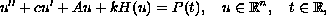whereand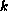are positive constants,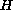is a globally Lipschitz function, and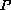is a bounded and continuous function.is a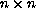symmetric matrix whose first eigenvalue is equal to zero and the others are positive. Under these conditions, we prove that for some values of, andthere exist a continuous manifold such that solutions starting in this manifold are exponentially bounded. Our results are applied to the spatial discretization of well-known second-order partial differential equations with Neumann boundary conditions.

Published October 24, 2000.
Math Subject Classifications: 34A34, 34C27, 34C30.
Key Words: center manifold, exponentially-bounded solutions.

Show me the PDF file (141K), TEX file, and other files for this article.Luis Garcia Universidad de los Andes Facultad de Ciencias Departamento de Matematica Merida 5101-Venezuela Hugo Leiva Universidad de los Andes Facultad de Ciencias Departamento de Matematica Merida 5101-Venezuela e-mail: hleiva@ula.ve